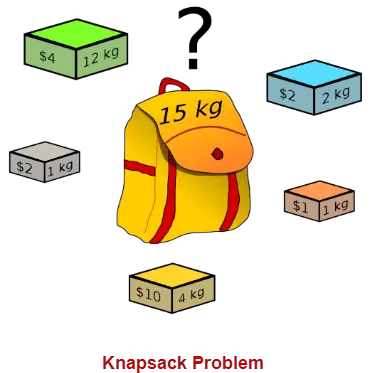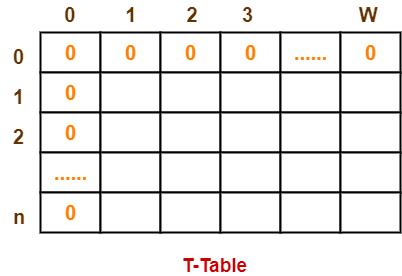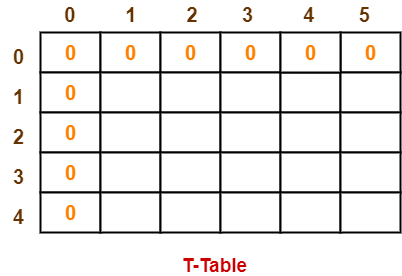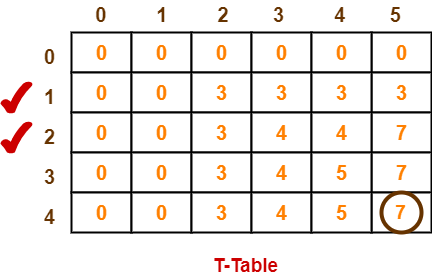Knapsack Problem-

You are given the following-

• A knapsack (kind of shoulder bag) with limited weight capacity.
• Few items each having some weight and value.

The problem states-

Which items should be placed into the knapsack such that-

• The value or profit obtained by putting the items into the knapsack is maximum.
• And the weight limit of the knapsack does not exceed.Knapsack Problem Variants-

Knapsack problem has the following two variants-

1. Fractional Knapsack Problem
2. 0/1 Knapsack Problem

0/1 Knapsack Problem-

In 0/1 Knapsack Problem,

• As the name suggests, items are indivisible here.
• We can not take the fraction of any item.
• We have to either take an item completely or leave it completely.
• It is solved using dynamic programming approach.

0/1 Knapsack Problem Using Dynamic Programming-

Consider-

• Knapsack weight capacity = w
• Number of items each having some weight and value = n

0/1 knapsack problem is solved using dynamic programming in the following steps-

Step-01:

• Draw a table say ‘T’ with (n+1) number of rows and (w+1) number of columns.
• Fill all the boxes of 0th row and 0th column with zeroes as shown-Step-02:

Start filling the table row wise top to bottom from left to right.

Use the following formula-

T (i , j) = max { T ( i-1 , j ) , valuei + T( i-1 , j – weighti ) }

Here, T(i , j) = maximum value of the selected items if we can take items 1 to i and have weight restrictions of j.

• This step leads to completely filling the table.
• Then, value of the last box represents the maximum possible value that can be put into the knapsack.

Step-03:

To identify the items that must be put into the knapsack to obtain that maximum profit,

• Consider the last column of the table.
• Start scanning the entries from bottom to top.
• On encountering an entry whose value is not same as the value stored in the entry immediately above it, mark the row label of that entry.
• After all the entries are scanned, the marked labels represent the items that must be put into the knapsack.

Time Complexity-

• Each entry of the table requires constant time θ(1) for its computation.
• It takes θ(nw) time to fill (n+1)(w+1) table entries.
• It takes θ(n) time for tracing the solution since tracing process traces the n rows.
• Thus, overall θ(nw) time is taken to solve 0/1 knapsack problem using dynamic programming.

Problem-

For the given set of items and knapsack capacity = 5 kg, find the optimal solution for the 0/1 knapsack problem making use of dynamic programming approach.

 Item Weight Value 1 2 3 2 3 4 3 4 5 4 5 6

OR

Find the optimal solution for the 0/1 knapsack problem making use of dynamic programming approach. Consider-

n = 4

w = 5 kg

(w1, w2, w3, w4) = (2, 3, 4, 5)

(b1, b2, b3, b4) = (3, 4, 5, 6)

OR

A thief enters a house for robbing it. He can carry a maximal weight of 5 kg into his bag. There are 4 items in the house with the following weights and values. What items should thief take if he either takes the item completely or leaves it completely?

 Item Weight (kg) Value (\$) Mirror 2 3 Silver nugget 3 4 Painting 4 5 Vase 5 6

Solution-

Given-

• Knapsack capacity (w) = 5 kg
• Number of items (n) = 4

Step-01:

• Draw a table say ‘T’ with (n+1) = 4 + 1 = 5 number of rows and (w+1) = 5 + 1 = 6 number of columns.
• Fill all the boxes of 0th row and 0th column with 0.Step-02:

Start filling the table row wise top to bottom from left to right using the formula-

T (i , j) = max { T ( i-1 , j ) , valuei + T( i-1 , j – weighti ) }

Finding T(1,1)-

We have,

• i = 1
• j = 1
• (value)i = (value)1 = 3
• (weight)i = (weight)1 = 2

Substituting the values, we get-

T(1,1) = max { T(1-1 , 1) , 3 + T(1-1 , 1-2) }

T(1,1) = max { T(0,1) , 3 + T(0,-1) }

T(1,1) = T(0,1) { Ignore T(0,-1) }

T(1,1) = 0

Finding T(1,2)-

We have,

• i = 1
• j = 2
• (value)i = (value)1 = 3
• (weight)i = (weight)1 = 2

Substituting the values, we get-

T(1,2) = max { T(1-1 , 2) , 3 + T(1-1 , 2-2) }

T(1,2) = max { T(0,2) , 3 + T(0,0) }

T(1,2) = max {0 , 3+0}

T(1,2) = 3

Finding T(1,3)-

We have,

• i = 1
• j = 3
• (value)i = (value)1 = 3
• (weight)i = (weight)1 = 2

Substituting the values, we get-

T(1,3) = max { T(1-1 , 3) , 3 + T(1-1 , 3-2) }

T(1,3) = max { T(0,3) , 3 + T(0,1) }

T(1,3) = max {0 , 3+0}

T(1,3) = 3

Finding T(1,4)-

We have,

• i = 1
• j = 4
• (value)i = (value)1 = 3
• (weight)i = (weight)1 = 2

Substituting the values, we get-

T(1,4) = max { T(1-1 , 4) , 3 + T(1-1 , 4-2) }

T(1,4) = max { T(0,4) , 3 + T(0,2) }

T(1,4) = max {0 , 3+0}

T(1,4) = 3

Finding T(1,5)-

We have,

• i = 1
• j = 5
• (value)i = (value)1 = 3
• (weight)i = (weight)1 = 2

Substituting the values, we get-

T(1,5) = max { T(1-1 , 5) , 3 + T(1-1 , 5-2) }

T(1,5) = max { T(0,5) , 3 + T(0,3) }

T(1,5) = max {0 , 3+0}

T(1,5) = 3

Finding T(2,1)-

We have,

• i = 2
• j = 1
• (value)i = (value)2 = 4
• (weight)i = (weight)2 = 3

Substituting the values, we get-

T(2,1) = max { T(2-1 , 1) , 4 + T(2-1 , 1-3) }

T(2,1) = max { T(1,1) , 4 + T(1,-2) }

T(2,1) = T(1,1) { Ignore T(1,-2) }

T(2,1) = 0

Finding T(2,2)-

We have,

• i = 2
• j = 2
• (value)i = (value)2 = 4
• (weight)i = (weight)2 = 3

Substituting the values, we get-

T(2,2) = max { T(2-1 , 2) , 4 + T(2-1 , 2-3) }

T(2,2) = max { T(1,2) , 4 + T(1,-1) }

T(2,2) = T(1,2) { Ignore T(1,-1) }

T(2,2) = 3

Finding T(2,3)-

We have,

• i = 2
• j = 3
• (value)i = (value)2 = 4
• (weight)i = (weight)2 = 3

Substituting the values, we get-

T(2,3) = max { T(2-1 , 3) , 4 + T(2-1 , 3-3) }

T(2,3) = max { T(1,3) , 4 + T(1,0) }

T(2,3) = max { 3 , 4+0 }

T(2,3) = 4

Finding T(2,4)-

We have,

• i = 2
• j = 4
• (value)i = (value)2 = 4
• (weight)i = (weight)2 = 3

Substituting the values, we get-

T(2,4) = max { T(2-1 , 4) , 4 + T(2-1 , 4-3) }

T(2,4) = max { T(1,4) , 4 + T(1,1) }

T(2,4) = max { 3 , 4+0 }

T(2,4) = 4

Finding T(2,5)-

We have,

• i = 2
• j = 5
• (value)i = (value)2 = 4
• (weight)i = (weight)2 = 3

Substituting the values, we get-

T(2,5) = max { T(2-1 , 5) , 4 + T(2-1 , 5-3) }

T(2,5) = max { T(1,5) , 4 + T(1,2) }

T(2,5) = max { 3 , 4+3 }

T(2,5) = 7

Similarly, compute all the entries.

After all the entries are computed and filled in the table, we get the following table-• The last entry represents the maximum possible value that can be put into the knapsack.
• So, maximum possible value that can be put into the knapsack = 7.

Identifying Items To Be Put Into Knapsack-

Following Step-04,

• We mark the rows labelled “1” and “2”.
• Thus, items that must be put into the knapsack to obtain the maximum value 7 are-

Item-1 and Item-2

To gain better understanding about 0/1 Knapsack Problem,

Watch this Video Lecture

Next Article- Travelling Salesman Problem

Get more notes and other study material of Design and Analysis of Algorithms.

Watch video lectures by visiting our YouTube channel LearnVidFun.

Knapsack Problem-

You are given the following-

• A knapsack (kind of shoulder bag) with limited weight capacity.
• Few items each having some weight and value.

The problem states-

Which items should be placed into the knapsack such that-

• The value or profit obtained by putting the items into the knapsack is maximum.
• And the weight limit of the knapsack does not exceed.Knapsack Problem Variants-

Knapsack problem has the following two variants-

1. Fractional Knapsack Problem
2. 0/1 Knapsack Problem

Fractional Knapsack Problem-

In Fractional Knapsack Problem,

• As the name suggests, items are divisible here.
• We can even put the fraction of any item into the knapsack if taking the complete item is not possible.
• It is solved using Greedy Method.

Fractional Knapsack Problem Using Greedy Method-

Fractional knapsack problem is solved using greedy method in the following steps-

Step-01:

For each item, compute its value / weight ratio.

Step-02:

Arrange all the items in decreasing order of their value / weight ratio.

Step-03:

Start putting the items into the knapsack beginning from the item with the highest ratio.

Put as many items as you can into the knapsack.

Time Complexity-

• The main time taking step is the sorting of all items in decreasing order of their value / weight ratio.
• If the items are already arranged in the required order, then while loop takes O(n) time.
• The average time complexity of Quick Sort is O(nlogn).
• Therefore, total time taken including the sort is O(nlogn).

Problem-

For the given set of items and knapsack capacity = 60 kg, find the optimal solution for the fractional knapsack problem making use of greedy approach.

 Item Weight Value 1 5 30 2 10 40 3 15 45 4 22 77 5 25 90

OR

Find the optimal solution for the fractional knapsack problem making use of greedy approach. Consider-

n = 5

w = 60 kg

(w1, w2, w3, w4, w5) = (5, 10, 15, 22, 25)

(b1, b2, b3, b4, b5) = (30, 40, 45, 77, 90)

OR

A thief enters a house for robbing it. He can carry a maximal weight of 60 kg into his bag. There are 5 items in the house with the following weights and values. What items should thief take if he can even take the fraction of any item with him?

 Item Weight Value 1 5 30 2 10 40 3 15 45 4 22 77 5 25 90

Solution-

Step-01:

Compute the value / weight ratio for each item-

 Items Weight Value Ratio 1 5 30 6 2 10 40 4 3 15 45 3 4 22 77 3.5 5 25 90 3.6

Step-02:

Sort all the items in decreasing order of their value / weight ratio-

I1 I2 I5 I4 I3

(6) (4) (3.6) (3.5) (3)

Step-03:

Start filling the knapsack by putting the items into it one by one.

 Knapsack Weight Items in Knapsack Cost 60 Ø 0 55 I1 30 45 I1, I2 70 20 I1, I2, I5 160

Now,

• Knapsack weight left to be filled is 20 kg but item-4 has a weight of 22 kg.
• Since in fractional knapsack problem, even the fraction of any item can be taken.
• So, knapsack will contain the following items-

< I1 , I2 , I5 , (20/22) I4 >

Total cost of the knapsack

= 160 + (20/27) x 77

= 160 + 70

= 230 units

Important Note-

Had the problem been a 0/1 knapsack problem, knapsack would contain the following items-

< I1 , I2 , I5 >

The knapsack’s total cost would be 160 units.

To gain better understanding about Fractional Knapsack Problem,

Watch this Video Lecture

Next Article- Job Sequencing With Deadlines

Get more notes and other study material of Design and Analysis of Algorithms.

Watch video lectures by visiting our YouTube channel LearnVidFun.